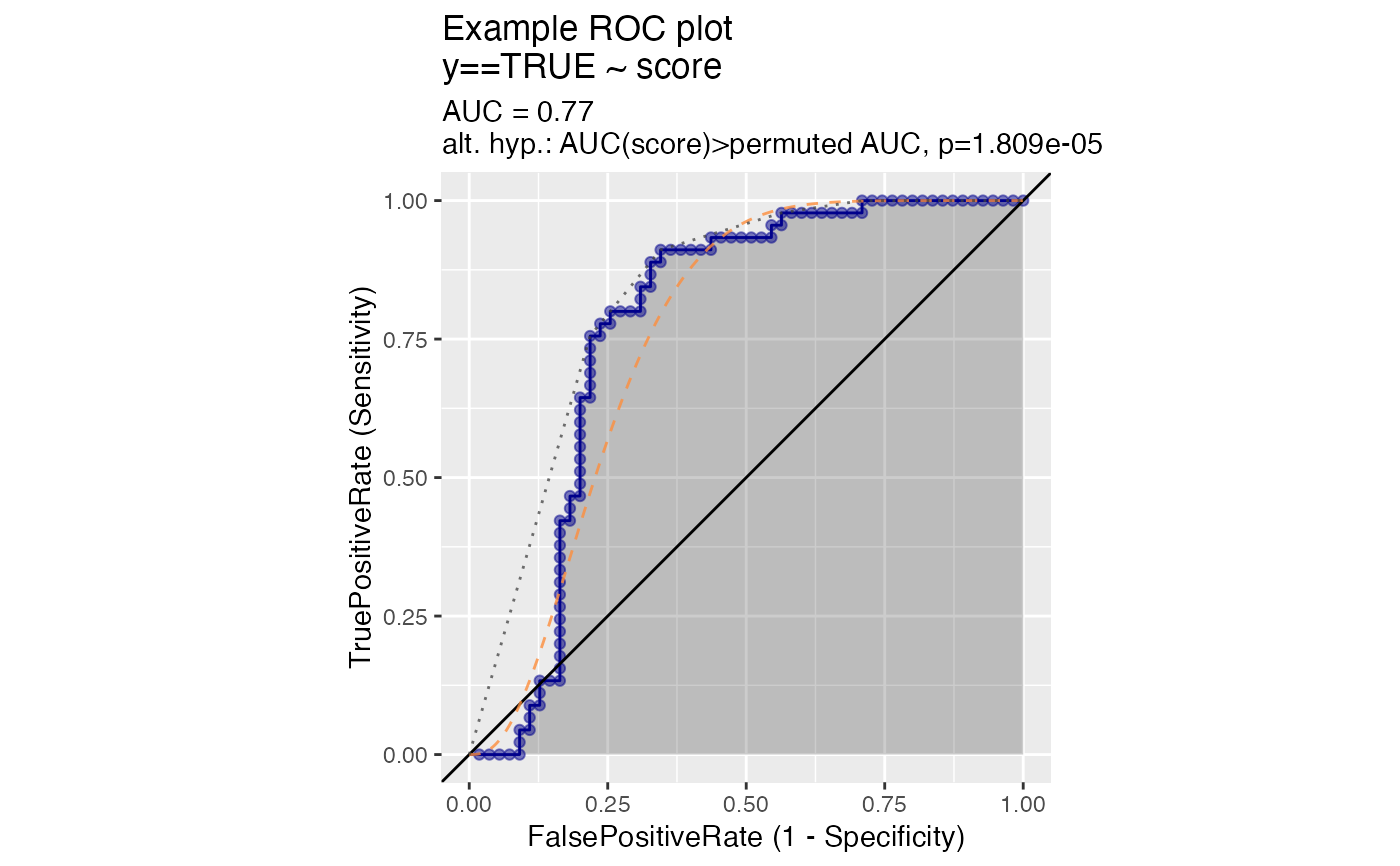ROCPlot(
frame,
xvar,
truthVar,
truthTarget,
title,
...,
estimate_sig = FALSE,
returnScores = FALSE,
nrep = 100,
parallelCluster = NULL,
curve_color = "darkblue",
fill_color = "black",
diag_color = "black",
beta_ideal_curve_color = "#fd8d3c",
beta1_ideal_curve_color = "#f03b20",
symmetric_ideal_curve_color = "#bd0026",
convex_hull_color = "#404040",
ideal_plot_step_size = 0.001
)

## Arguments

frame data frame to get values from name of the independent (input or model) column in frame name of the dependent (output or result to be modeled) column in frame value we consider to be positive title to place on plot no unnamed argument, added to force named binding of later arguments. logical, if TRUE estimate and display significance of difference from AUC 0.5. logical if TRUE return detailed permutedScores number of permutation repetitions to estimate p values. (optional) a cluster object created by package parallel or package snow. color of the ROC curve shading color for the area under the curve color for the AUC=0.5 line (x=y) logical, if TRUE add the beta(a, b), beta(c, d) ideal curve found by moment matching. color for ideal curve. logical, if TRUE add the beta(1, a), beta(b, 2) ideal curve defined in https://journals.sagepub.com/doi/abs/10.1177/0272989X15582210 color for ideal curve. logical, if TRUE add the ideal curve as discussed in https://win-vector.com/2020/09/13/why-working-with-auc-is-more-powerful-than-one-might-think/. color for ideal curve. logical, if TRUE add convex hull to plot color for convex hull curve step size used in ideal plots

## Details

See https://www.nature.com/articles/nmeth.3945 for a discussion of true positive and false positive rates, and how the ROC plot relates to the precision/recall plot.

PRTPlot, ThresholdPlot

## Examples


beta_example <- function(
n,
shape1_pos, shape2_pos,
shape1_neg, shape2_neg) {
d <- data.frame(
y = sample(
c(TRUE, FALSE),
size = n,
replace = TRUE),
score = 0.0
)
d$score[d$y] <- rbeta(sum(d$y), shape1 = shape1_pos, shape2 = shape2_pos) d$score[!d$y] <- rbeta(sum(!d$y), shape1 = shape1_neg, shape2 = shape2_neg)
d
}

d1 <- beta_example(
100,
shape1_pos = 6,
shape2_pos = 5,
shape1_neg = 1,
shape2_neg = 2)

ROCPlot(
d1,
xvar = "score",
truthVar = "y", truthTarget = TRUE,
title="Example ROC plot",
estimate_sig = TRUE,